# FIN Chapter 5_V1.ppt

May. 29, 2023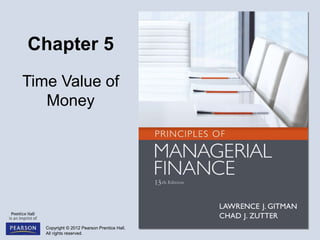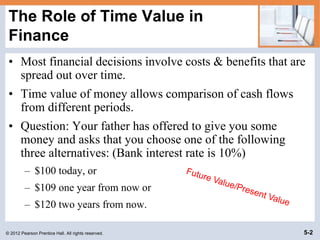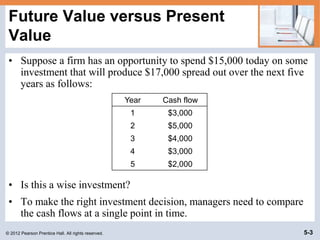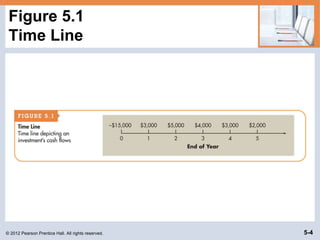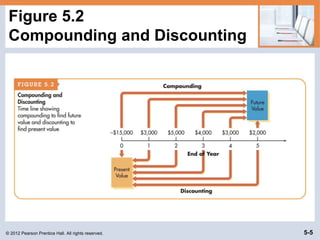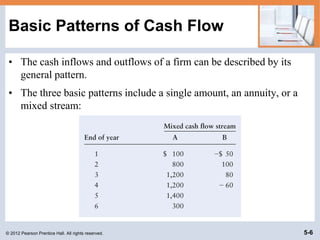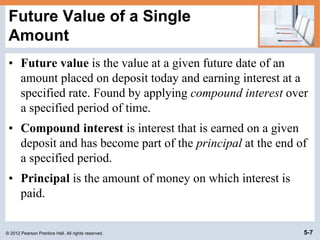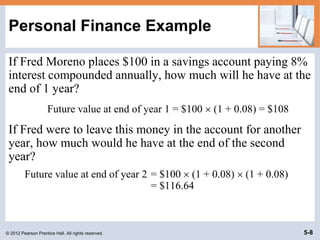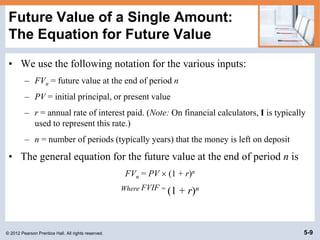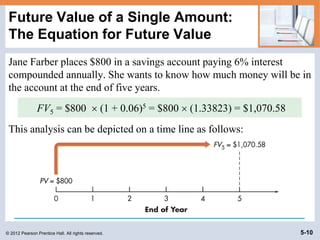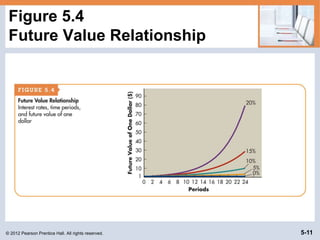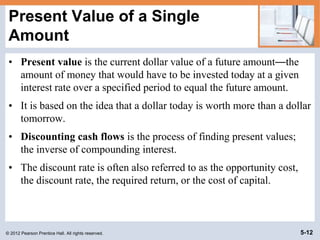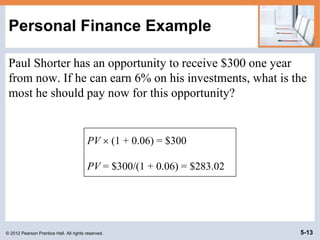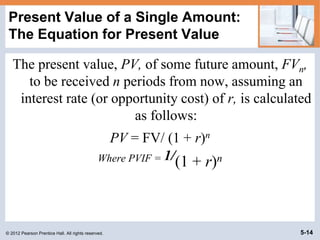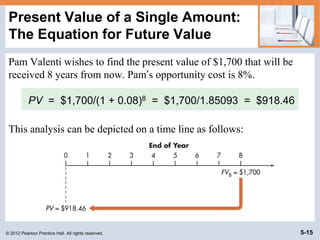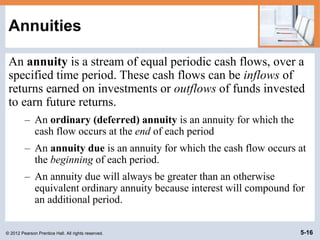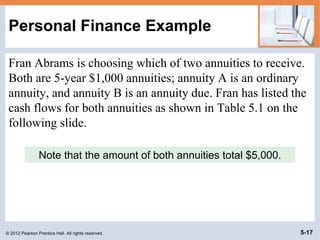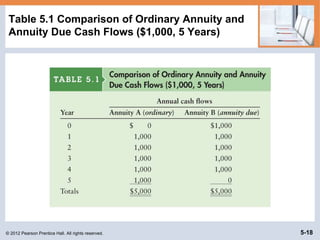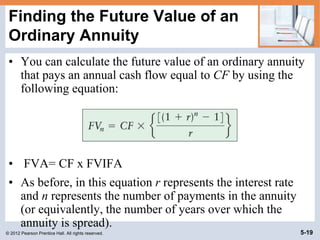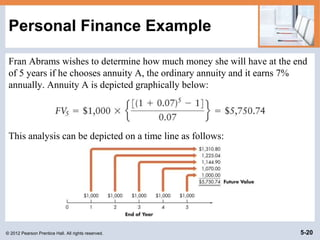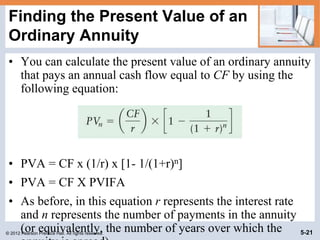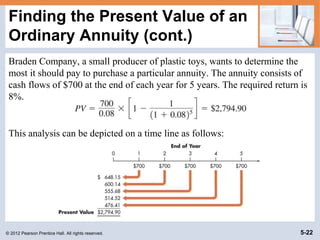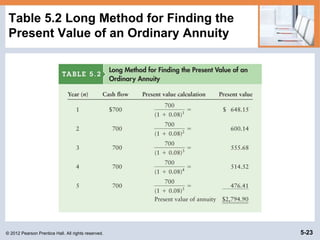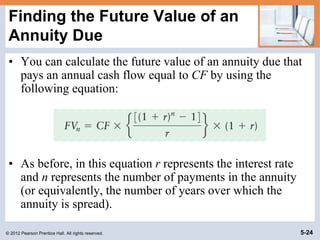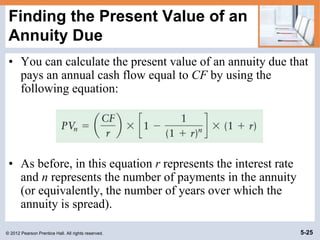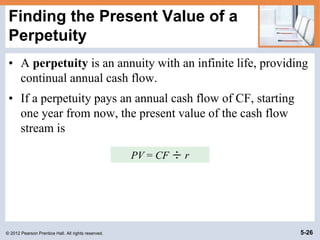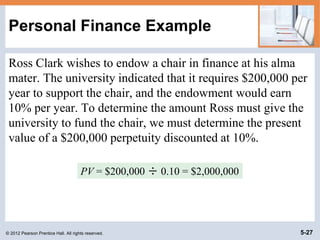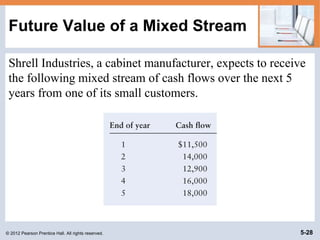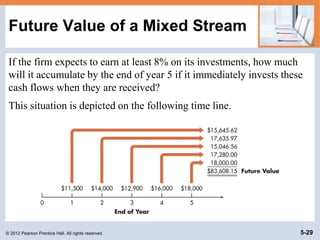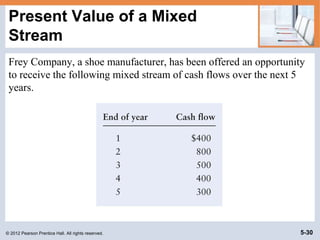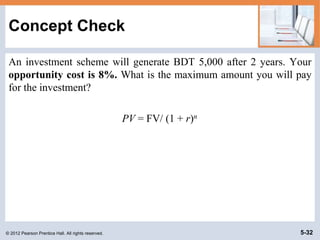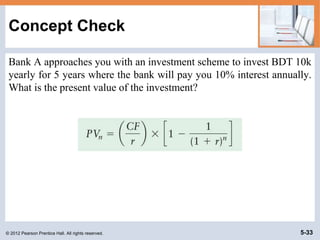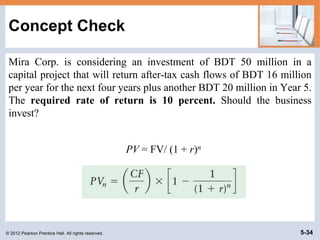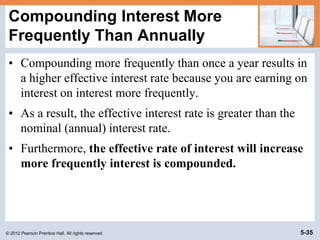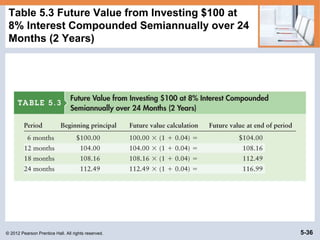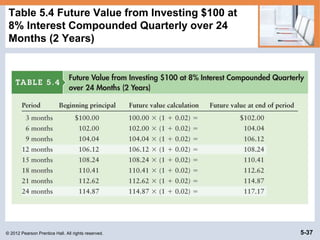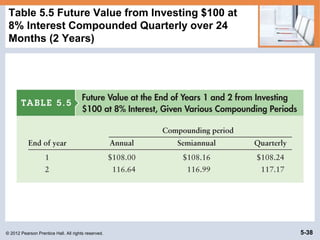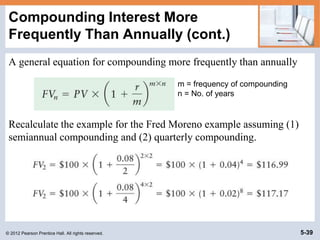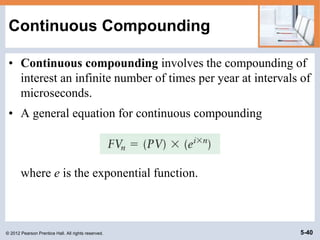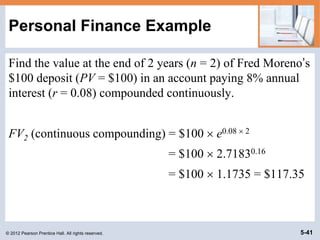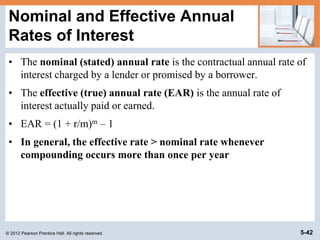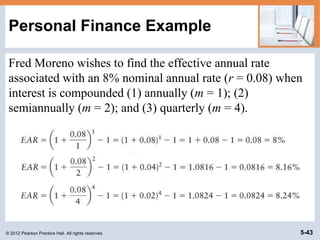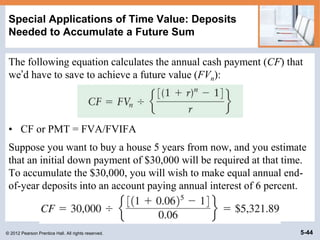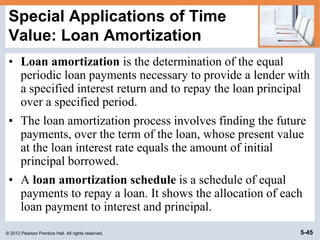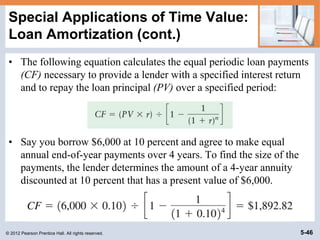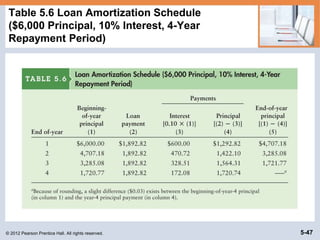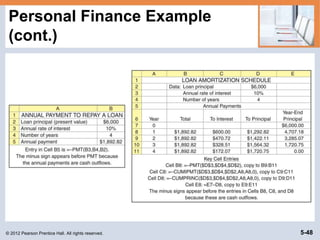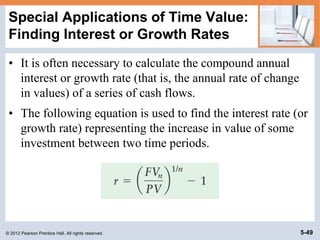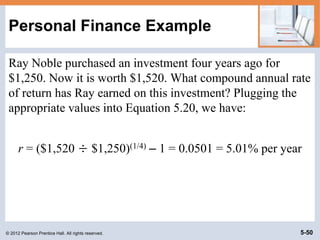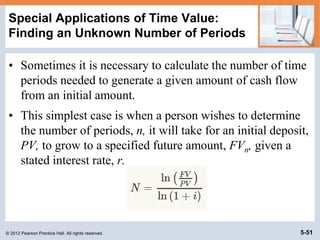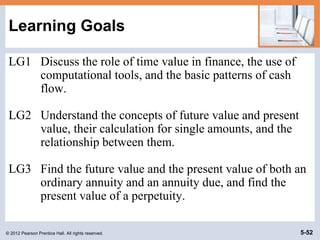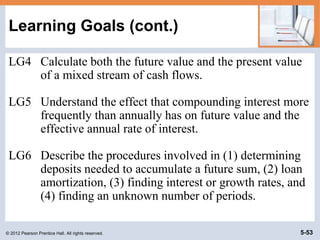1 of 53

### FIN Chapter 5_V1.ppt

• 2. © 2012 Pearson Prentice Hall. All rights reserved. 5-2 The Role of Time Value in Finance • Most financial decisions involve costs & benefits that are spread out over time. • Time value of money allows comparison of cash flows from different periods. • Question: Your father has offered to give you some money and asks that you choose one of the following three alternatives: (Bank interest rate is 10%) – \$100 today, or – \$109 one year from now or – \$120 two years from now.
• 3. © 2012 Pearson Prentice Hall. All rights reserved. 5-3 Future Value versus Present Value • Suppose a firm has an opportunity to spend \$15,000 today on some investment that will produce \$17,000 spread out over the next five years as follows: • Is this a wise investment? • To make the right investment decision, managers need to compare the cash flows at a single point in time. Year Cash flow 1 \$3,000 2 \$5,000 3 \$4,000 4 \$3,000 5 \$2,000
• 6. © 2012 Pearson Prentice Hall. All rights reserved. 5-6 Basic Patterns of Cash Flow • The cash inflows and outflows of a firm can be described by its general pattern. • The three basic patterns include a single amount, an annuity, or a mixed stream:
• 7. © 2012 Pearson Prentice Hall. All rights reserved. 5-7 Future Value of a Single Amount • Future value is the value at a given future date of an amount placed on deposit today and earning interest at a specified rate. Found by applying compound interest over a specified period of time. • Compound interest is interest that is earned on a given deposit and has become part of the principal at the end of a specified period. • Principal is the amount of money on which interest is paid.
• 8. © 2012 Pearson Prentice Hall. All rights reserved. 5-8 Personal Finance Example If Fred Moreno places \$100 in a savings account paying 8% interest compounded annually, how much will he have at the end of 1 year? Future value at end of year 1 = \$100  (1 + 0.08) = \$108 If Fred were to leave this money in the account for another year, how much would he have at the end of the second year? Future value at end of year 2 = \$100  (1 + 0.08)  (1 + 0.08) = \$116.64
• 9. © 2012 Pearson Prentice Hall. All rights reserved. 5-9 Future Value of a Single Amount: The Equation for Future Value • We use the following notation for the various inputs: – FVn = future value at the end of period n – PV = initial principal, or present value – r = annual rate of interest paid. (Note: On financial calculators, I is typically used to represent this rate.) – n = number of periods (typically years) that the money is left on deposit • The general equation for the future value at the end of period n is FVn = PV  (1 + r)n Where FVIF = (1 + r)n
• 10. © 2012 Pearson Prentice Hall. All rights reserved. 5-10 Future Value of a Single Amount: The Equation for Future Value Jane Farber places \$800 in a savings account paying 6% interest compounded annually. She wants to know how much money will be in the account at the end of five years. This analysis can be depicted on a time line as follows: FV5 = \$800  (1 + 0.06)5 = \$800  (1.33823) = \$1,070.58
• 12. © 2012 Pearson Prentice Hall. All rights reserved. 5-12 Present Value of a Single Amount • Present value is the current dollar value of a future amount—the amount of money that would have to be invested today at a given interest rate over a specified period to equal the future amount. • It is based on the idea that a dollar today is worth more than a dollar tomorrow. • Discounting cash flows is the process of finding present values; the inverse of compounding interest. • The discount rate is often also referred to as the opportunity cost, the discount rate, the required return, or the cost of capital.
• 13. © 2012 Pearson Prentice Hall. All rights reserved. 5-13 Personal Finance Example Paul Shorter has an opportunity to receive \$300 one year from now. If he can earn 6% on his investments, what is the most he should pay now for this opportunity? PV  (1 + 0.06) = \$300 PV = \$300/(1 + 0.06) = \$283.02
• 14. © 2012 Pearson Prentice Hall. All rights reserved. 5-14 Present Value of a Single Amount: The Equation for Present Value The present value, PV, of some future amount, FVn, to be received n periods from now, assuming an interest rate (or opportunity cost) of r, is calculated as follows: PV = FV/ (1 + r)n Where PVIF = 1/(1 + r)n
• 15. © 2012 Pearson Prentice Hall. All rights reserved. 5-15 Present Value of a Single Amount: The Equation for Future Value Pam Valenti wishes to find the present value of \$1,700 that will be received 8 years from now. Pam’s opportunity cost is 8%. This analysis can be depicted on a time line as follows: PV = \$1,700/(1 + 0.08)8 = \$1,700/1.85093 = \$918.46
• 16. © 2012 Pearson Prentice Hall. All rights reserved. 5-16 Annuities An annuity is a stream of equal periodic cash flows, over a specified time period. These cash flows can be inflows of returns earned on investments or outflows of funds invested to earn future returns. – An ordinary (deferred) annuity is an annuity for which the cash flow occurs at the end of each period – An annuity due is an annuity for which the cash flow occurs at the beginning of each period. – An annuity due will always be greater than an otherwise equivalent ordinary annuity because interest will compound for an additional period.
• 17. © 2012 Pearson Prentice Hall. All rights reserved. 5-17 Personal Finance Example Fran Abrams is choosing which of two annuities to receive. Both are 5-year \$1,000 annuities; annuity A is an ordinary annuity, and annuity B is an annuity due. Fran has listed the cash flows for both annuities as shown in Table 5.1 on the following slide. Note that the amount of both annuities total \$5,000.
• 18. © 2012 Pearson Prentice Hall. All rights reserved. 5-18 Table 5.1 Comparison of Ordinary Annuity and Annuity Due Cash Flows (\$1,000, 5 Years)
• 19. © 2012 Pearson Prentice Hall. All rights reserved. 5-19 Finding the Future Value of an Ordinary Annuity • You can calculate the future value of an ordinary annuity that pays an annual cash flow equal to CF by using the following equation: • FVA= CF x FVIFA • As before, in this equation r represents the interest rate and n represents the number of payments in the annuity (or equivalently, the number of years over which the annuity is spread).
• 20. © 2012 Pearson Prentice Hall. All rights reserved. 5-20 Personal Finance Example Fran Abrams wishes to determine how much money she will have at the end of 5 years if he chooses annuity A, the ordinary annuity and it earns 7% annually. Annuity A is depicted graphically below: This analysis can be depicted on a time line as follows:
• 21. © 2012 Pearson Prentice Hall. All rights reserved. 5-21 Finding the Present Value of an Ordinary Annuity • You can calculate the present value of an ordinary annuity that pays an annual cash flow equal to CF by using the following equation: • PVA = CF x (1/r) x [1- 1/(1+r)n] • PVA = CF X PVIFA • As before, in this equation r represents the interest rate and n represents the number of payments in the annuity (or equivalently, the number of years over which the
• 22. © 2012 Pearson Prentice Hall. All rights reserved. 5-22 Finding the Present Value of an Ordinary Annuity (cont.) Braden Company, a small producer of plastic toys, wants to determine the most it should pay to purchase a particular annuity. The annuity consists of cash flows of \$700 at the end of each year for 5 years. The required return is 8%. This analysis can be depicted on a time line as follows:
• 23. © 2012 Pearson Prentice Hall. All rights reserved. 5-23 Table 5.2 Long Method for Finding the Present Value of an Ordinary Annuity
• 24. © 2012 Pearson Prentice Hall. All rights reserved. 5-24 Finding the Future Value of an Annuity Due • You can calculate the future value of an annuity due that pays an annual cash flow equal to CF by using the following equation: • As before, in this equation r represents the interest rate and n represents the number of payments in the annuity (or equivalently, the number of years over which the annuity is spread).
• 25. © 2012 Pearson Prentice Hall. All rights reserved. 5-25 Finding the Present Value of an Annuity Due • You can calculate the present value of an annuity due that pays an annual cash flow equal to CF by using the following equation: • As before, in this equation r represents the interest rate and n represents the number of payments in the annuity (or equivalently, the number of years over which the annuity is spread).
• 26. © 2012 Pearson Prentice Hall. All rights reserved. 5-26 Finding the Present Value of a Perpetuity • A perpetuity is an annuity with an infinite life, providing continual annual cash flow. • If a perpetuity pays an annual cash flow of CF, starting one year from now, the present value of the cash flow stream is PV = CF ÷ r
• 27. © 2012 Pearson Prentice Hall. All rights reserved. 5-27 Personal Finance Example Ross Clark wishes to endow a chair in finance at his alma mater. The university indicated that it requires \$200,000 per year to support the chair, and the endowment would earn 10% per year. To determine the amount Ross must give the university to fund the chair, we must determine the present value of a \$200,000 perpetuity discounted at 10%. PV = \$200,000 ÷ 0.10 = \$2,000,000
• 28. © 2012 Pearson Prentice Hall. All rights reserved. 5-28 Future Value of a Mixed Stream Shrell Industries, a cabinet manufacturer, expects to receive the following mixed stream of cash flows over the next 5 years from one of its small customers.
• 29. © 2012 Pearson Prentice Hall. All rights reserved. 5-29 Future Value of a Mixed Stream If the firm expects to earn at least 8% on its investments, how much will it accumulate by the end of year 5 if it immediately invests these cash flows when they are received? This situation is depicted on the following time line.
• 30. © 2012 Pearson Prentice Hall. All rights reserved. 5-30 Present Value of a Mixed Stream Frey Company, a shoe manufacturer, has been offered an opportunity to receive the following mixed stream of cash flows over the next 5 years.
• 31. © 2012 Pearson Prentice Hall. All rights reserved. 5-31 Present Value of a Mixed Stream If the firm must earn at least 9% on its investments, what is the most it should pay for this opportunity? This situation is depicted on the following time line.
• 32. © 2012 Pearson Prentice Hall. All rights reserved. 5-32 Concept Check An investment scheme will generate BDT 5,000 after 2 years. Your opportunity cost is 8%. What is the maximum amount you will pay for the investment? PV = FV/ (1 + r)n
• 33. © 2012 Pearson Prentice Hall. All rights reserved. 5-33 Concept Check Bank A approaches you with an investment scheme to invest BDT 10k yearly for 5 years where the bank will pay you 10% interest annually. What is the present value of the investment?
• 34. © 2012 Pearson Prentice Hall. All rights reserved. 5-34 Concept Check Mira Corp. is considering an investment of BDT 50 million in a capital project that will return after-tax cash flows of BDT 16 million per year for the next four years plus another BDT 20 million in Year 5. The required rate of return is 10 percent. Should the business invest? PV = FV/ (1 + r)n
• 35. © 2012 Pearson Prentice Hall. All rights reserved. 5-35 Compounding Interest More Frequently Than Annually • Compounding more frequently than once a year results in a higher effective interest rate because you are earning on interest on interest more frequently. • As a result, the effective interest rate is greater than the nominal (annual) interest rate. • Furthermore, the effective rate of interest will increase more frequently interest is compounded.
• 36. © 2012 Pearson Prentice Hall. All rights reserved. 5-36 Table 5.3 Future Value from Investing \$100 at 8% Interest Compounded Semiannually over 24 Months (2 Years)
• 37. © 2012 Pearson Prentice Hall. All rights reserved. 5-37 Table 5.4 Future Value from Investing \$100 at 8% Interest Compounded Quarterly over 24 Months (2 Years)
• 38. © 2012 Pearson Prentice Hall. All rights reserved. 5-38 Table 5.5 Future Value from Investing \$100 at 8% Interest Compounded Quarterly over 24 Months (2 Years)
• 39. © 2012 Pearson Prentice Hall. All rights reserved. 5-39 Compounding Interest More Frequently Than Annually (cont.) A general equation for compounding more frequently than annually Recalculate the example for the Fred Moreno example assuming (1) semiannual compounding and (2) quarterly compounding. m = frequency of compounding n = No. of years
• 40. © 2012 Pearson Prentice Hall. All rights reserved. 5-40 Continuous Compounding • Continuous compounding involves the compounding of interest an infinite number of times per year at intervals of microseconds. • A general equation for continuous compounding where e is the exponential function.
• 41. © 2012 Pearson Prentice Hall. All rights reserved. 5-41 Personal Finance Example Find the value at the end of 2 years (n = 2) of Fred Moreno’s \$100 deposit (PV = \$100) in an account paying 8% annual interest (r = 0.08) compounded continuously. FV2 (continuous compounding) = \$100  e0.08  2 = \$100  2.71830.16 = \$100  1.1735 = \$117.35
• 42. © 2012 Pearson Prentice Hall. All rights reserved. 5-42 Nominal and Effective Annual Rates of Interest • The nominal (stated) annual rate is the contractual annual rate of interest charged by a lender or promised by a borrower. • The effective (true) annual rate (EAR) is the annual rate of interest actually paid or earned. • EAR = (1 + r/m)m – 1 • In general, the effective rate > nominal rate whenever compounding occurs more than once per year
• 43. © 2012 Pearson Prentice Hall. All rights reserved. 5-43 Personal Finance Example Fred Moreno wishes to find the effective annual rate associated with an 8% nominal annual rate (r = 0.08) when interest is compounded (1) annually (m = 1); (2) semiannually (m = 2); and (3) quarterly (m = 4).
• 44. © 2012 Pearson Prentice Hall. All rights reserved. 5-44 Special Applications of Time Value: Deposits Needed to Accumulate a Future Sum The following equation calculates the annual cash payment (CF) that we’d have to save to achieve a future value (FVn): • CF or PMT = FVA/FVIFA Suppose you want to buy a house 5 years from now, and you estimate that an initial down payment of \$30,000 will be required at that time. To accumulate the \$30,000, you will wish to make equal annual end- of-year deposits into an account paying annual interest of 6 percent.
• 45. © 2012 Pearson Prentice Hall. All rights reserved. 5-45 Special Applications of Time Value: Loan Amortization • Loan amortization is the determination of the equal periodic loan payments necessary to provide a lender with a specified interest return and to repay the loan principal over a specified period. • The loan amortization process involves finding the future payments, over the term of the loan, whose present value at the loan interest rate equals the amount of initial principal borrowed. • A loan amortization schedule is a schedule of equal payments to repay a loan. It shows the allocation of each loan payment to interest and principal.
• 46. © 2012 Pearson Prentice Hall. All rights reserved. 5-46 Special Applications of Time Value: Loan Amortization (cont.) • The following equation calculates the equal periodic loan payments (CF) necessary to provide a lender with a specified interest return and to repay the loan principal (PV) over a specified period: • Say you borrow \$6,000 at 10 percent and agree to make equal annual end-of-year payments over 4 years. To find the size of the payments, the lender determines the amount of a 4-year annuity discounted at 10 percent that has a present value of \$6,000.
• 47. © 2012 Pearson Prentice Hall. All rights reserved. 5-47 Table 5.6 Loan Amortization Schedule (\$6,000 Principal, 10% Interest, 4-Year Repayment Period)